# KS 4 Mathematics A 6 Quadratic equations 1

• Slides: 48KS 4 Mathematics A 6 Quadratic equations 1 of 48 © Boardworks Ltd 2005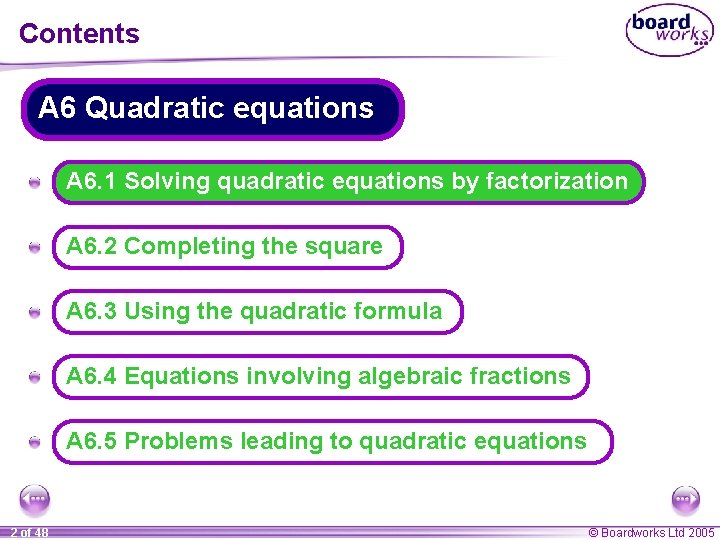Contents A 6 Quadratic equations A A 6. 1 Solving quadratic equations by factorization A A 6. 2 Completing the square A A 6. 3 Using the quadratic formula A A 6. 4 Equations involving algebraic fractions A A 6. 5 Problems leading to quadratic equations 2 of 48 © Boardworks Ltd 2005Find the width of the rectangle The length of a rectangle is 4 cm more than its width. The area of the rectangle is 45 cm 2. Find the width of the rectangle. If we call the width of the rectangle x we can draw the following diagram: x+4 x Using the information about the area of the rectangle we can write an equation: x(x + 4) = 45 3 of 48 © Boardworks Ltd 2005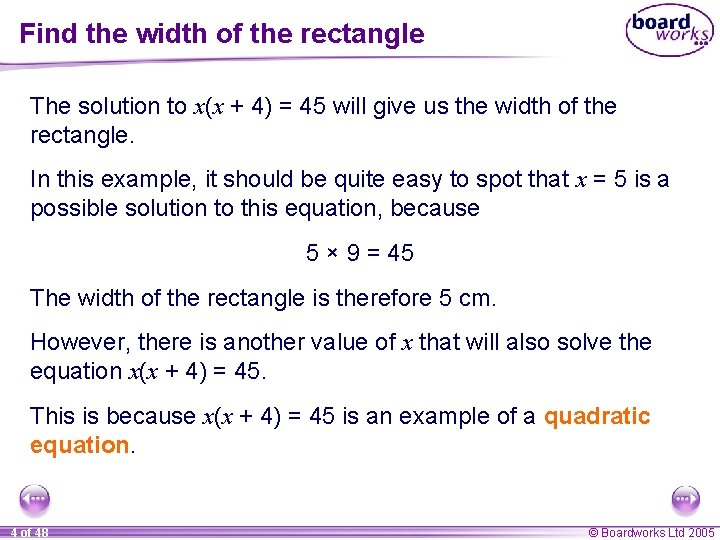Find the width of the rectangle The solution to x(x + 4) = 45 will give us the width of the rectangle. In this example, it should be quite easy to spot that x = 5 is a possible solution to this equation, because 5 × 9 = 45 The width of the rectangle is therefore 5 cm. However, there is another value of x that will also solve the equation x(x + 4) = 45. This is because x(x + 4) = 45 is an example of a quadratic equation. 4 of 48 © Boardworks Ltd 2005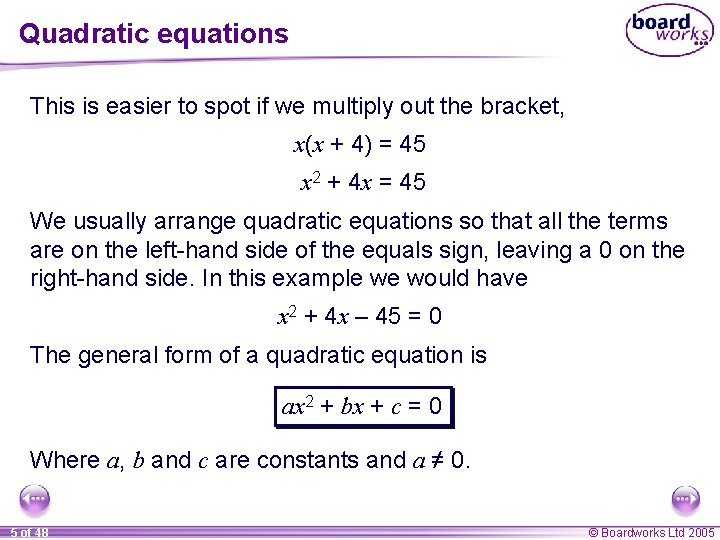Quadratic equations This is easier to spot if we multiply out the bracket, x(x + 4) = 45 x 2 + 4 x = 45 We usually arrange quadratic equations so that all the terms are on the left-hand side of the equals sign, leaving a 0 on the right-hand side. In this example we would have x 2 + 4 x – 45 = 0 The general form of a quadratic equation is ax 2 + bx + c = 0 Where a, b and c are constants and a ≠ 0. 5 of 48 © Boardworks Ltd 2005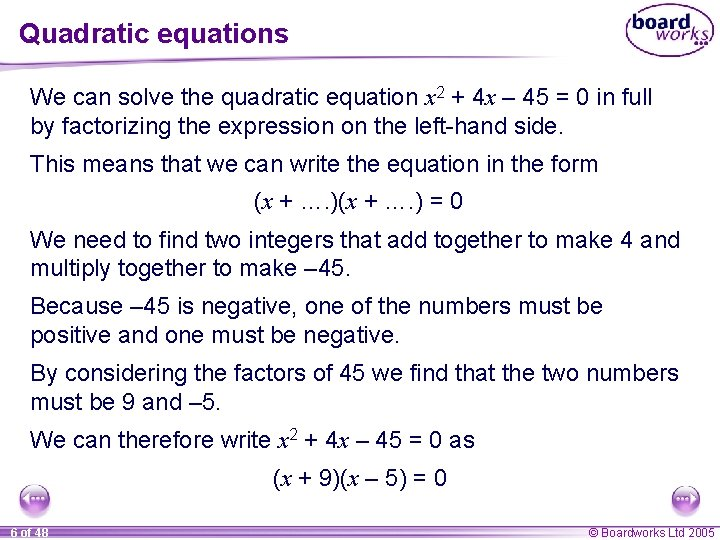Quadratic equations We can solve the quadratic equation x 2 + 4 x – 45 = 0 in full by factorizing the expression on the left-hand side. This means that we can write the equation in the form (x + …. ) = 0 We need to find two integers that add together to make 4 and multiply together to make – 45. Because – 45 is negative, one of the numbers must be positive and one must be negative. By considering the factors of 45 we find that the two numbers must be 9 and – 5. We can therefore write x 2 + 4 x – 45 = 0 as (x + 9)(x – 5) = 0 6 of 48 © Boardworks Ltd 2005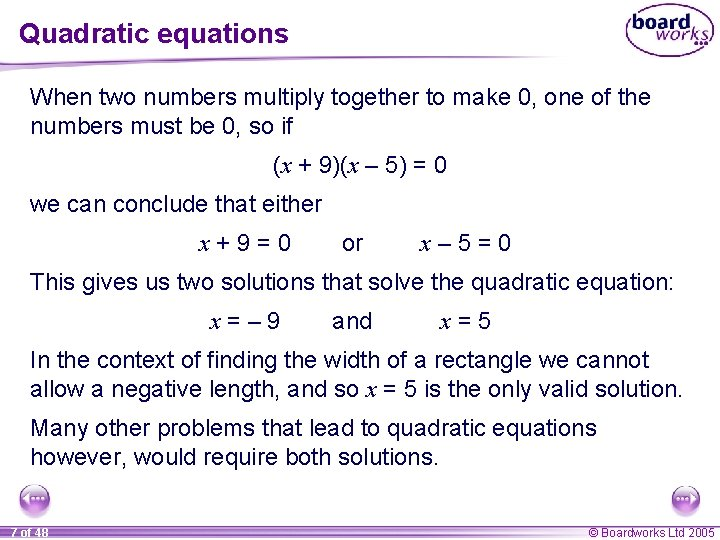Quadratic equations When two numbers multiply together to make 0, one of the numbers must be 0, so if (x + 9)(x – 5) = 0 we can conclude that either x+9=0 or x– 5=0 This gives us two solutions that solve the quadratic equation: x=– 9 and x=5 In the context of finding the width of a rectangle we cannot allow a negative length, and so x = 5 is the only valid solution. Many other problems that lead to quadratic equations however, would require both solutions. 7 of 48 © Boardworks Ltd 2005Solving quadratic equations by factorization Solve the equation x 2 = 3 x by factorization. Start by rearranging the equation so that the terms are on the left-hand side, x 2 – 3 x = 0 Factorizing the left-hand side gives us x(x – 3) = 0 So x=0 or x– 3=0 x=3 8 of 48 © Boardworks Ltd 2005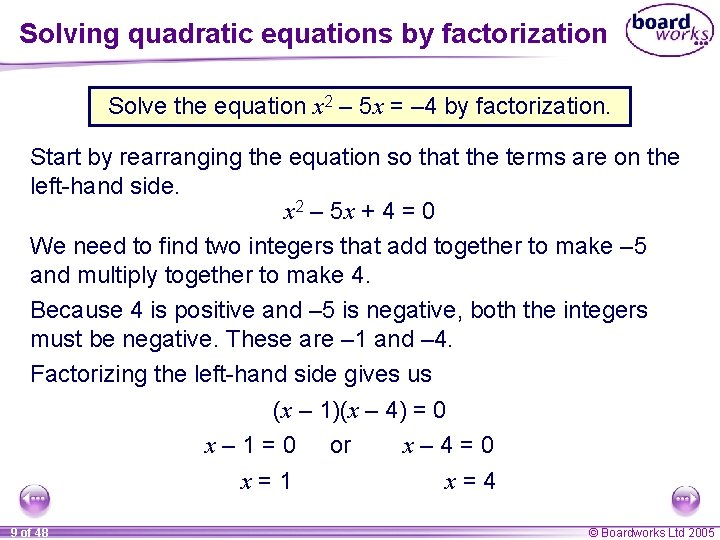Solving quadratic equations by factorization Solve the equation x 2 – 5 x = – 4 by factorization. Start by rearranging the equation so that the terms are on the left-hand side. x 2 – 5 x + 4 = 0 We need to find two integers that add together to make – 5 and multiply together to make 4. Because 4 is positive and – 5 is negative, both the integers must be negative. These are – 1 and – 4. Factorizing the left-hand side gives us (x – 1)(x – 4) = 0 x – 1 = 0 or x– 4=0 x=1 x=4 9 of 48 © Boardworks Ltd 2005Solving quadratic equations by factorization 10 of 48 © Boardworks Ltd 2005Demonstrating solutions using graphs 11 of 48 © Boardworks Ltd 2005Contents A 6 Quadratic equations A A 6. 1 Solving quadratic equations by factorization A A 6. 2 Completing the square A A 6. 3 Using the quadratic formula A A 6. 4 Equations involving algebraic fractions A A 6. 5 Problems leading to quadratic equations 12 of 48 © Boardworks Ltd 2005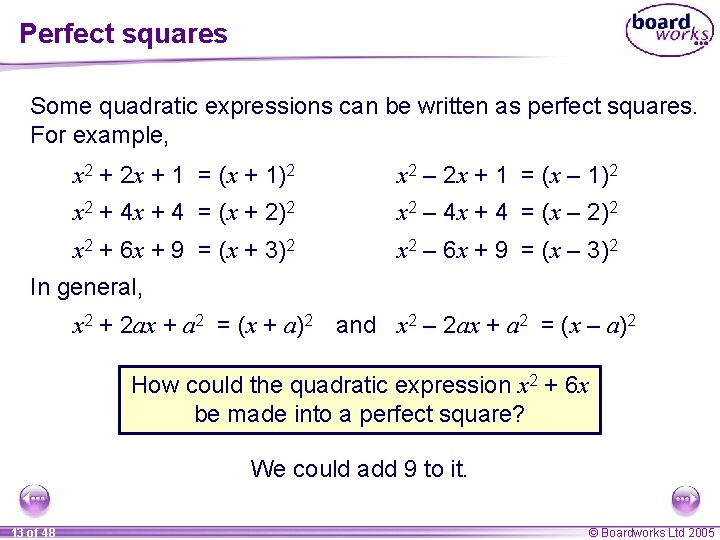Perfect squares Some quadratic expressions can be written as perfect squares. For example, x 2 + 2 x + 1 = (x + 1)2 x 2 – 2 x + 1 = (x – 1)2 x 2 + 4 x + 4 = (x + 2)2 x 2 – 4 x + 4 = (x – 2)2 x 2 + 6 x + 9 = (x + 3)2 x 2 – 6 x + 9 = (x – 3)2 In general, x 2 + 2 ax + a 2 = (x + a)2 and x 2 – 2 ax + a 2 = (x – a)2 How could the quadratic expression x 2 + 6 x be made into a perfect square? We could add 9 to it. 13 of 48 © Boardworks Ltd 2005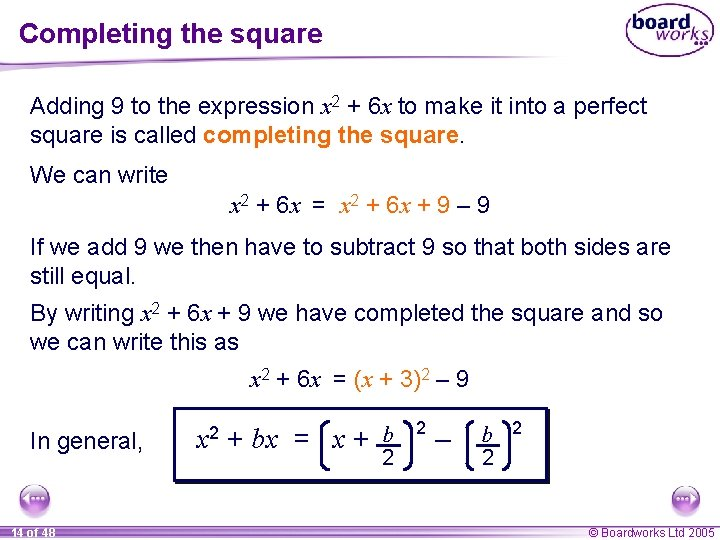Completing the square Adding 9 to the expression x 2 + 6 x to make it into a perfect square is called completing the square. We can write x 2 + 6 x = x 2 + 6 x + 9 – 9 If we add 9 we then have to subtract 9 so that both sides are still equal. By writing x 2 + 6 x + 9 we have completed the square and so we can write this as x 2 + 6 x = (x + 3)2 – 9 In general, 14 of 48 x 2 + bx = x + b 2 2 – b 2 2 © Boardworks Ltd 2005Completing the square Complete the square for x 2 – 10 x. Compare this expression to (x – 5)2 = x 2 – 10 x + 25 x 2 – 10 x = x 2 – 10 x + 25 – 25 = (x – 5)2 – 25 Complete the square for x 2 – 3 x. Compare this expression to (x – 1. 5)2 = x 2 – 3 x + 2. 25 x 2 – 3 x = x 2 – 3 x + 2. 25 – 2. 25 = (x + 1. 5)2 – 2. 25 15 of 48 © Boardworks Ltd 2005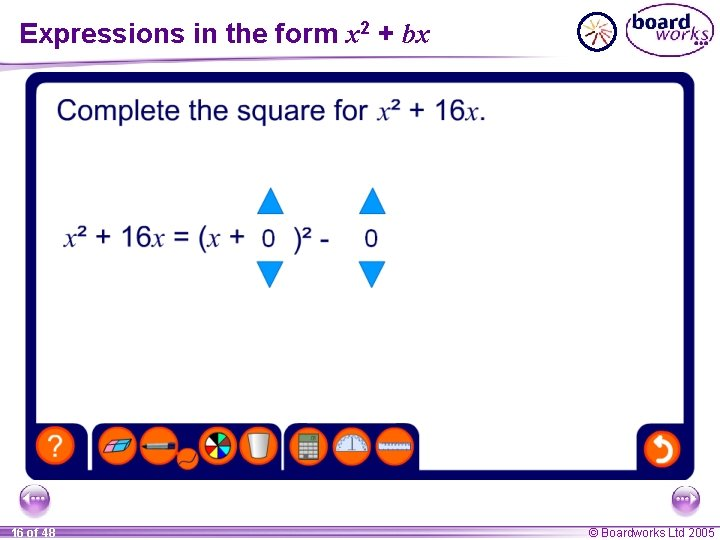Expressions in the form x 2 + bx 16 of 48 © Boardworks Ltd 2005Completing the square How can we complete the square for x 2 + 8 x + 9? Look at the coefficient of x. This is 8 so compare the expression to (x + 4)2 = x 2 + 8 x + 16. x 2 + 8 x + 9 = x 2 + 8 x + 16 – 16 + 9 In general, = (x + 4)2 – 7 x 2 + bx + c = x + 17 of 48 b 2 2 – b 2 2 +c © Boardworks Ltd 2005Completing the square Complete the square for x 2 + 12 x – 5. Compare this expression to (x + 6)2 = x 2 + 12 x + 36 x 2 + 12 x – 5 = x 2 + 12 x + 36 – 5 = (x 2 + 6) – 41 Complete the square for x 2 – 5 x + 16 Compare this expression to (x – 2. 5)2 = x 2 – 5 x + 6. 25 x 2 – 5 x + 16 = x 2 – 5 x + 6. 25 – 6. 25 + 16 = (x 2 – 2. 5) + 9. 75 18 of 48 © Boardworks Ltd 2005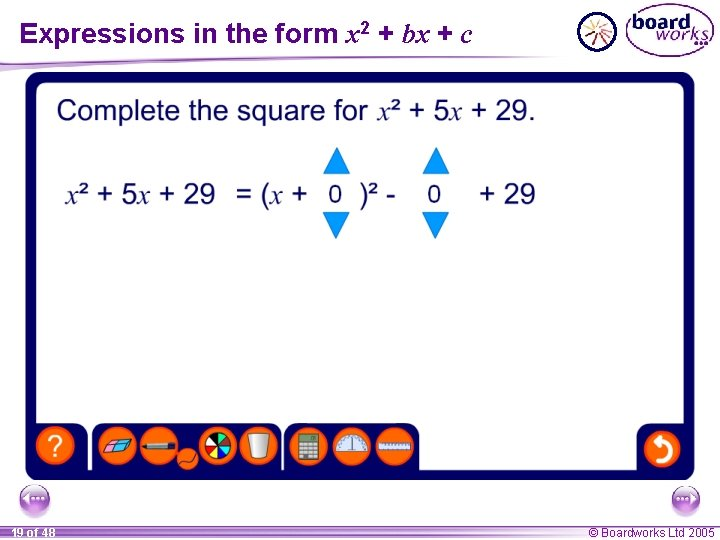Expressions in the form x 2 + bx + c 19 of 48 © Boardworks Ltd 2005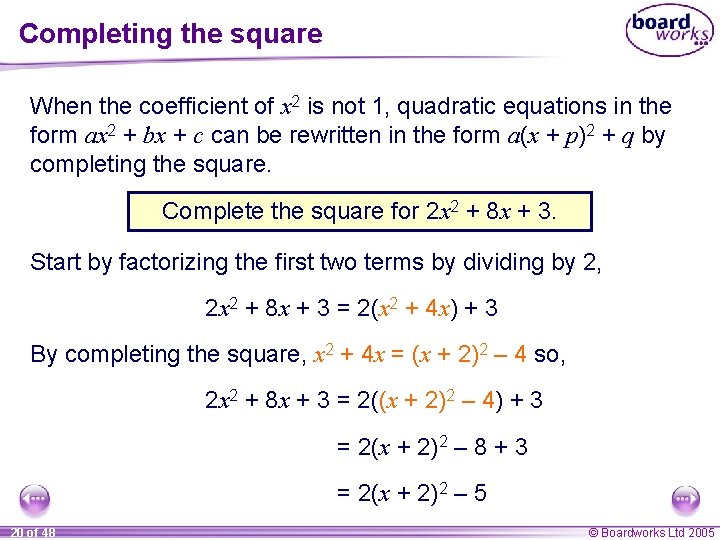Completing the square When the coefficient of x 2 is not 1, quadratic equations in the form ax 2 + bx + c can be rewritten in the form a(x + p)2 + q by completing the square. Complete the square for 2 x 2 + 8 x + 3. Start by factorizing the first two terms by dividing by 2, 2 x 2 + 8 x + 3 = 2(x 2 + 4 x) + 3 By completing the square, x 2 + 4 x = (x + 2)2 – 4 so, 2 x 2 + 8 x + 3 = 2((x + 2)2 – 4) + 3 = 2(x + 2)2 – 8 + 3 = 2(x + 2)2 – 5 20 of 48 © Boardworks Ltd 2005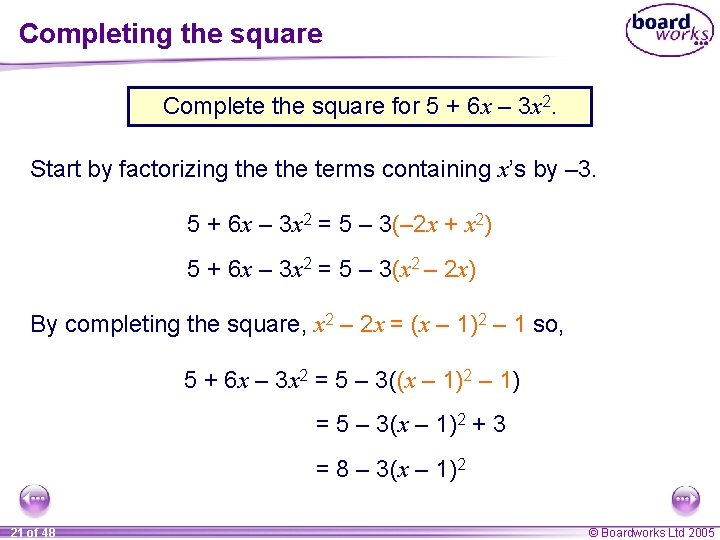Completing the square Complete the square for 5 + 6 x – 3 x 2. Start by factorizing the terms containing x’s by – 3. 5 + 6 x – 3 x 2 = 5 – 3(– 2 x + x 2) 5 + 6 x – 3 x 2 = 5 – 3(x 2 – 2 x) By completing the square, x 2 – 2 x = (x – 1)2 – 1 so, 5 + 6 x – 3 x 2 = 5 – 3((x – 1)2 – 1) = 5 – 3(x – 1)2 + 3 = 8 – 3(x – 1)2 21 of 48 © Boardworks Ltd 2005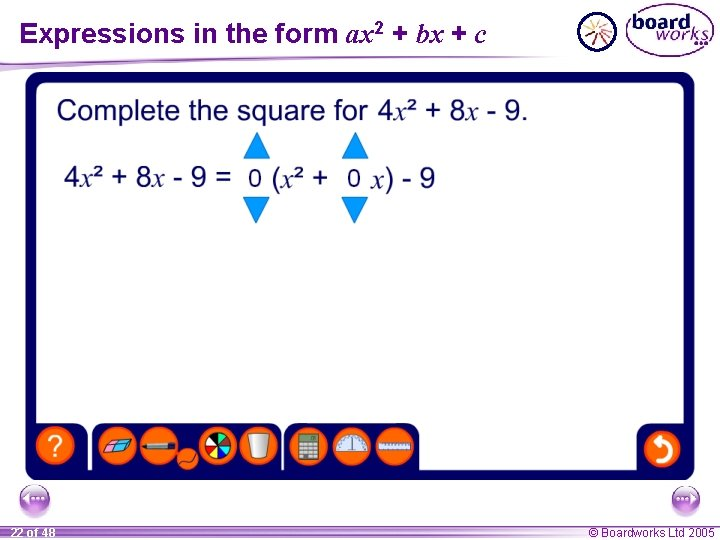Expressions in the form ax 2 + bx + c 22 of 48 © Boardworks Ltd 2005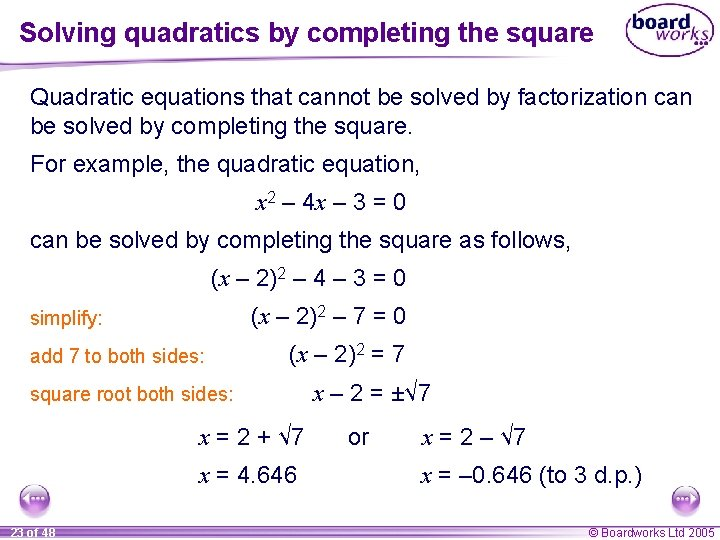Solving quadratics by completing the square Quadratic equations that cannot be solved by factorization can be solved by completing the square. For example, the quadratic equation, x 2 – 4 x – 3 = 0 can be solved by completing the square as follows, (x – 2)2 – 4 – 3 = 0 (x – 2)2 – 7 = 0 simplify: add 7 to both sides: (x – 2)2 = 7 square root both sides: x = 2 + √ 7 x = 4. 646 23 of 48 x – 2 = ±√ 7 or x = 2 – √ 7 x = – 0. 646 (to 3 d. p. ) © Boardworks Ltd 2005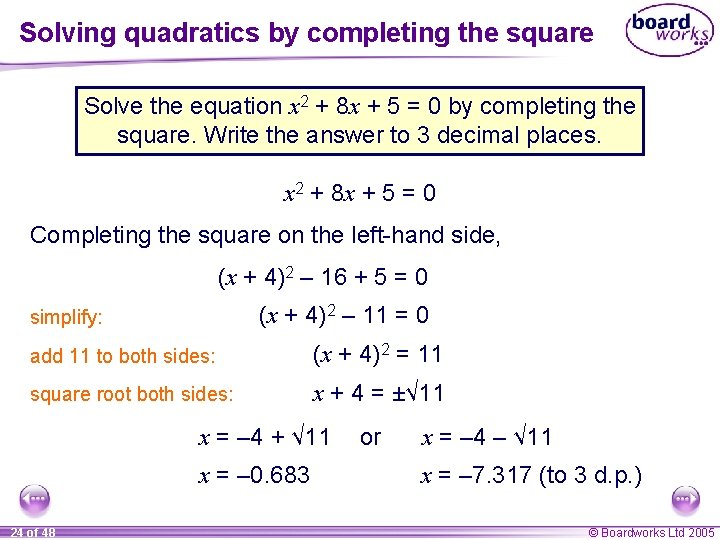Solving quadratics by completing the square Solve the equation x 2 + 8 x + 5 = 0 by completing the square. Write the answer to 3 decimal places. x 2 + 8 x + 5 = 0 Completing the square on the left-hand side, (x + 4)2 – 16 + 5 = 0 (x + 4)2 – 11 = 0 simplify: add 11 to both sides: (x + 4)2 = 11 square root both sides: x + 4 = ±√ 11 x = – 4 + √ 11 x = – 0. 683 24 of 48 or x = – 4 – √ 11 x = – 7. 317 (to 3 d. p. ) © Boardworks Ltd 2005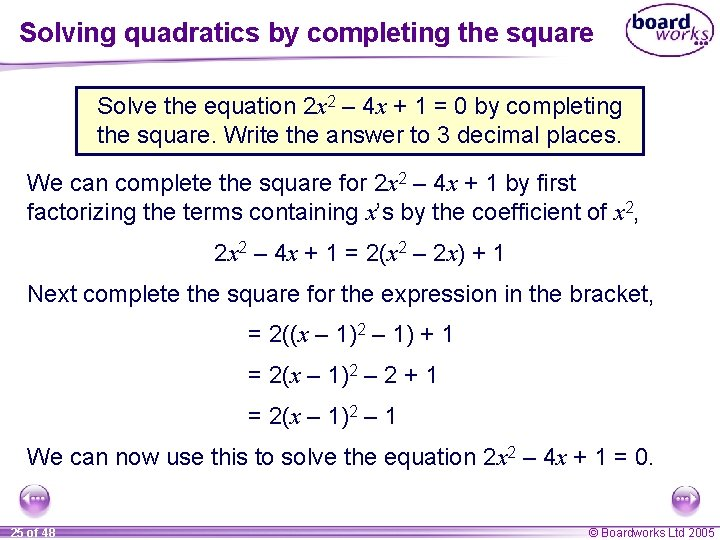Solving quadratics by completing the square Solve the equation 2 x 2 – 4 x + 1 = 0 by completing the square. Write the answer to 3 decimal places. We can complete the square for 2 x 2 – 4 x + 1 by first factorizing the terms containing x’s by the coefficient of x 2, 2 x 2 – 4 x + 1 = 2(x 2 – 2 x) + 1 Next complete the square for the expression in the bracket, = 2((x – 1)2 – 1) + 1 = 2(x – 1)2 – 2 + 1 = 2(x – 1)2 – 1 We can now use this to solve the equation 2 x 2 – 4 x + 1 = 0. 25 of 48 © Boardworks Ltd 2005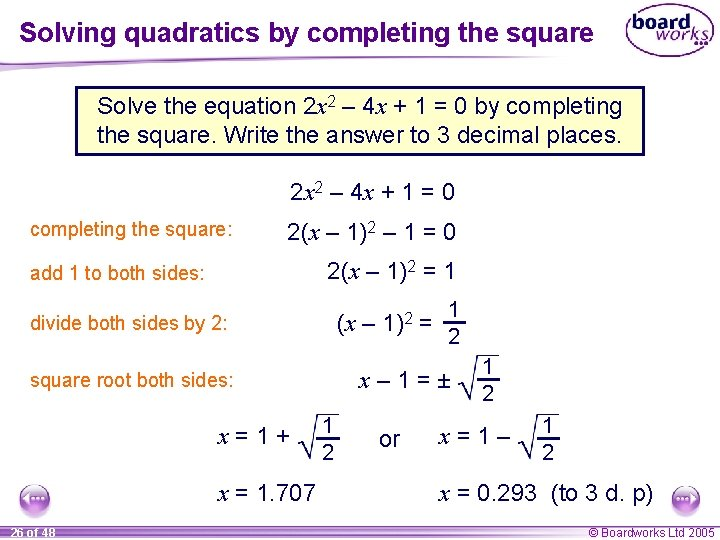Solving quadratics by completing the square Solve the equation 2 x 2 – 4 x + 1 = 0 by completing the square. Write the answer to 3 decimal places. 2 x 2 – 4 x + 1 = 0 completing the square: 2(x – 1)2 – 1 = 0 2(x – 1)2 = 1 add 1 to both sides: (x – 1)2 = divide both sides by 2: x– 1=± square root both sides: x=1+ x = 1. 707 26 of 48 1 2 or 1 2 x=1– 1 2 x = 0. 293 (to 3 d. p) © Boardworks Ltd 2005Contents A 6 Quadratic equations A A 6. 1 Solving quadratic equations by factorization A A 6. 2 Completing the square A A 6. 3 Using the quadratic formula A A 6. 4 Equations involving algebraic fractions A A 6. 5 Problems leading to quadratic equations 27 of 48 © Boardworks Ltd 2005Using the quadratic formula Any quadratic equation of the form, ax 2 + bx + c = 0 can be solved by substituting the values of a, b and c into the formula, –b ± b 2 – 4 ac x= 2 a This equation can be derived by completing the square on the general form of the quadratic equation. 28 of 48 © Boardworks Ltd 2005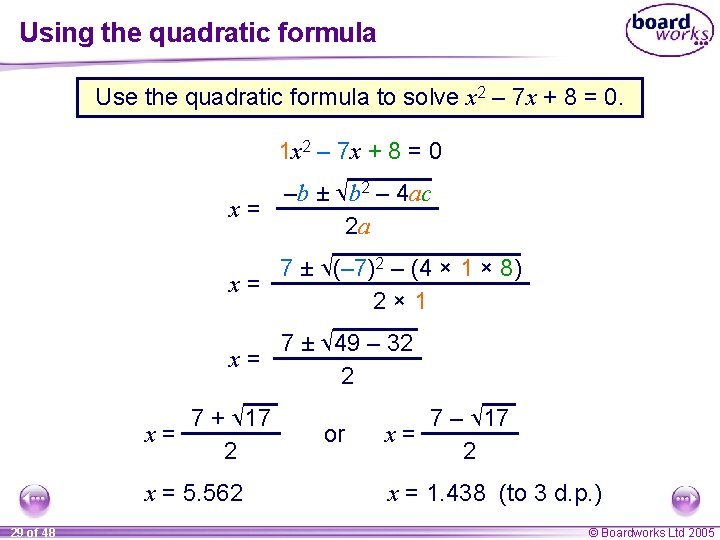Using the quadratic formula Use the quadratic formula to solve x 2 – 7 x + 8 = 0. 1 x 2 – 7 x + 8 = 0 –b ± b 2 – 4 ac x= 2 a 7 ± (– 7)2 – (4 × 1 × 8) x= 2× 1 7 ± 49 – 32 x= 2 7 + 17 x= 2 x = 5. 562 29 of 48 or 7 – 17 x= 2 x = 1. 438 (to 3 d. p. ) © Boardworks Ltd 2005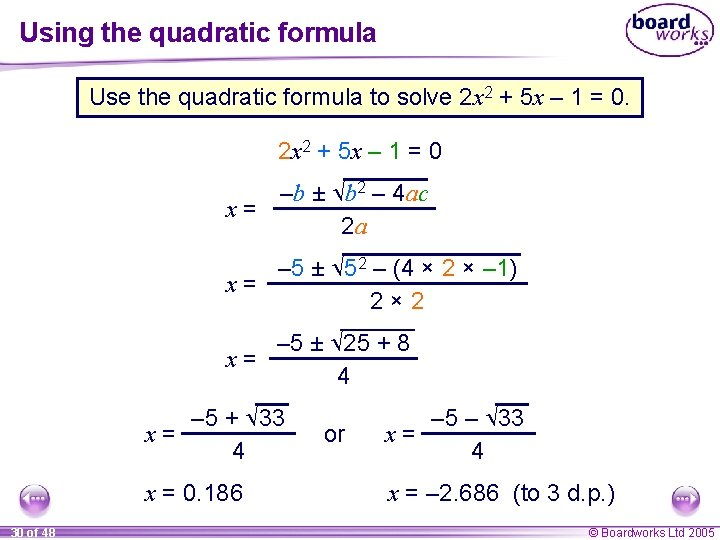Using the quadratic formula Use the quadratic formula to solve 2 x 2 + 5 x – 1 = 0 –b ± b 2 – 4 ac x= 2 a – 5 ± 52 – (4 × 2 × – 1) x= 2× 2 – 5 ± 25 + 8 x= 4 – 5 + 33 x= 4 x = 0. 186 30 of 48 or – 5 – 33 x= 4 x = – 2. 686 (to 3 d. p. ) © Boardworks Ltd 2005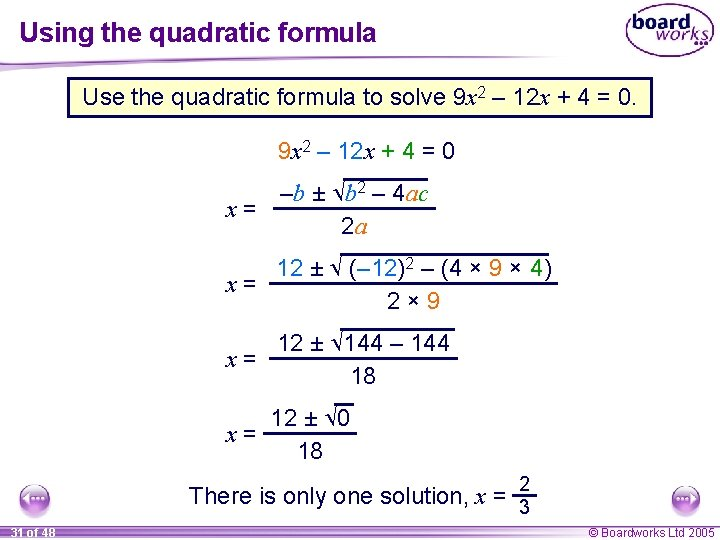Using the quadratic formula Use the quadratic formula to solve 9 x 2 – 12 x + 4 = 0 –b ± b 2 – 4 ac x= 2 a 12 ± (– 12)2 – (4 × 9 × 4) x= 2× 9 12 ± 144 – 144 x= 18 12 ± 0 x= 18 2 There is only one solution, x = 3 31 of 48 © Boardworks Ltd 2005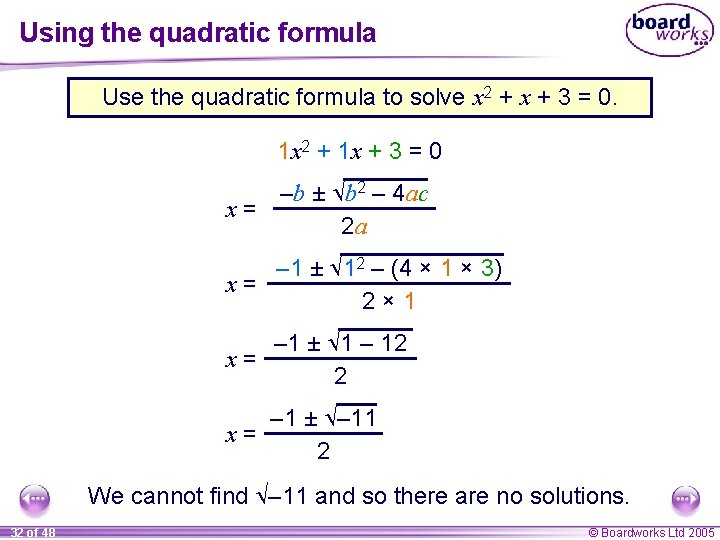Using the quadratic formula Use the quadratic formula to solve x 2 + x + 3 = 0. 1 x 2 + 1 x + 3 = 0 –b ± b 2 – 4 ac x= 2 a – 1 ± 12 – (4 × 1 × 3) x= 2× 1 – 1 ± 1 – 12 x= 2 – 1 ± – 11 x= 2 We cannot find – 11 and so there are no solutions. 32 of 48 © Boardworks Ltd 2005Using b 2 – 4 ac From using the quadratic formula, –b ± b 2 – 4 ac x= 2 a we can see that we can use the expression under the square root sign, b 2 – 4 ac, to decide how many solutions there are. When b 2 – 4 ac is positive, there are two solutions. When b 2 – 4 ac is equal to zero, there is one solution. When b 2 – 4 ac is negative, there are no solutions. 33 of 48 © Boardworks Ltd 2005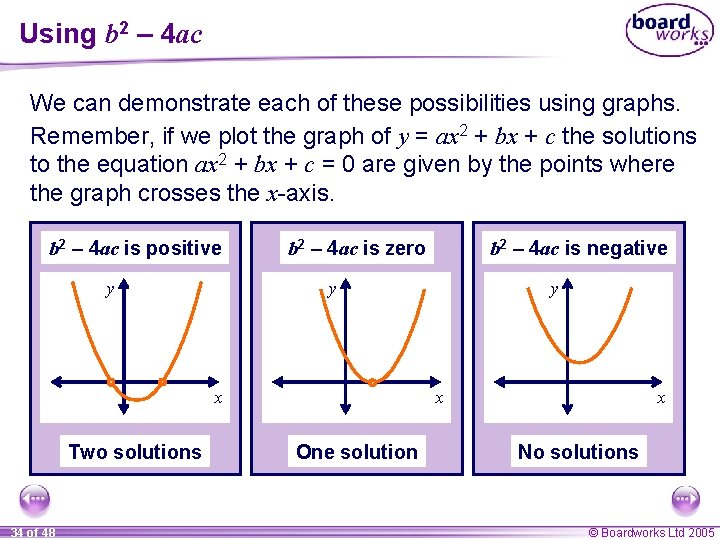Using b 2 – 4 ac We can demonstrate each of these possibilities using graphs. Remember, if we plot the graph of y = ax 2 + bx + c the solutions to the equation ax 2 + bx + c = 0 are given by the points where the graph crosses the x-axis. b 2 – 4 ac is positive y b 2 – 4 ac is zero y x Two solutions 34 of 48 b 2 – 4 ac is negative y x One solution x No solutions © Boardworks Ltd 2005Using b 2 – 4 ac 35 of 48 © Boardworks Ltd 2005Contents A 6 Quadratic equations A A 6. 1 Solving quadratic equations by factorization A A 6. 2 Completing the square A A 6. 3 Using the quadratic formula A A 6. 4 Equations involving algebraic fractions A A 6. 5 Problems leading to quadratic equations 36 of 48 © Boardworks Ltd 2005Equations involving algebraic fractions Some equations involving algebraic fractions rearrange to give quadratic equations. For example: 1 5 + = 2 x x+4 The first step when solving equations involving fractions is to multiply through by the product of the denominators. multiply by x(x + 4): x + 4 + 5 x = 2 x(x + 4) expand brackets and simplify: 6 x + 4 = 2 x 2 + 8 x collect all terms on the r. h. s. : 0 = 2 x 2 + 2 x – 4 divide by 2: 0 = x 2 + x – 2 factorize: 0 = (x + 2)(x – 1) x = – 2 37 of 48 or x=1 © Boardworks Ltd 2005Equations involving algebraic fractions Solve 4 3 – = 1 x+2 x+8 Start by multiplying through by x + 2 and x + 8 to remove the denominators, 4(x + 8) – 3(x + 2) = (x + 2)(x + 8) expand the brackets: 4 x + 32 – 3 x – 6 = x 2 + 10 x + 16 x + 26 = x 2 + 10 x + 16 simplify: collect all terms on the r. h. s. : 0 = x 2 + 9 x – 10 factorize: 0 = (x + 10)(x – 1) x = – 10 38 of 48 or x=1 © Boardworks Ltd 2005Equations involving algebraic fractions may also lead to quadratic equations that do not factorize. For example, x 2 – = 3 4–x x Solve, Multiply through by x(4 – x): expand the brackets: collect terms on the l. h. s. : divide by 2: x 2 + 2(4 – x) = 3 x(4 – x) x 2 + 8 – 2 x = 12 x – 3 x 2 4 x 2 – 14 x + 8 = 0 2 x 2 – 7 x + 4 = 0 This quadratic equation cannot be solved by factorization so we have to solve it using the quadratic formula. 39 of 48 © Boardworks Ltd 2005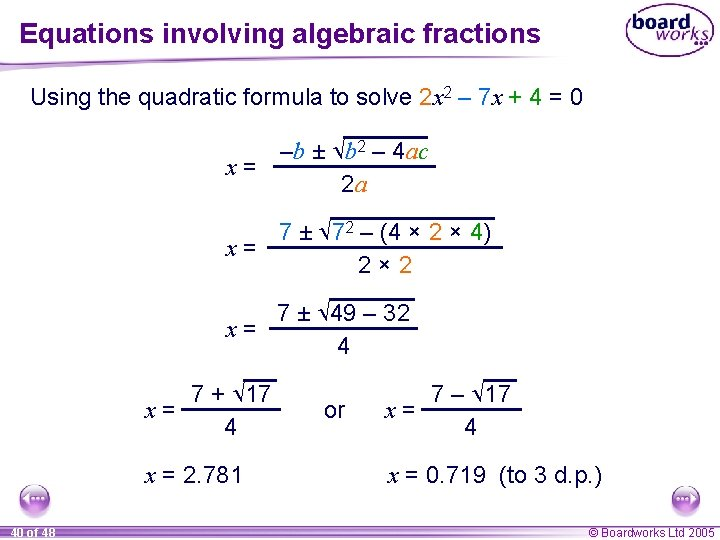Equations involving algebraic fractions Using the quadratic formula to solve 2 x 2 – 7 x + 4 = 0 –b ± b 2 – 4 ac x= 2 a 7 ± 72 – (4 × 2 × 4) x= 2× 2 7 ± 49 – 32 x= 4 7 + 17 x= 4 x = 2. 781 40 of 48 or 7 – 17 x= 4 x = 0. 719 (to 3 d. p. ) © Boardworks Ltd 2005Contents A 6 Quadratic equations A A 6. 1 Solving quadratic equations by factorization A A 6. 2 Completing the square A A 6. 3 Using the quadratic formula A A 6. 4 Equations involving algebraic fractions A A 6. 5 Problems leading to quadratic equations 41 of 48 © Boardworks Ltd 2005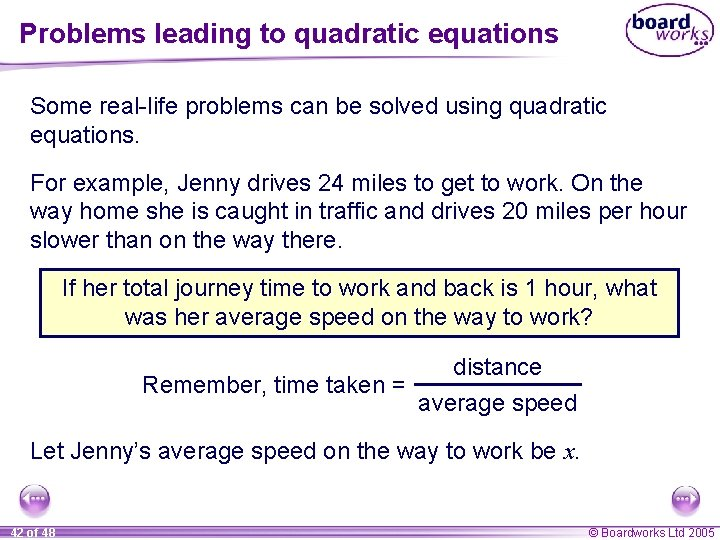Problems leading to quadratic equations Some real-life problems can be solved using quadratic equations. For example, Jenny drives 24 miles to get to work. On the way home she is caught in traffic and drives 20 miles per hour slower than on the way there. If her total journey time to work and back is 1 hour, what was her average speed on the way to work? distance Remember, time taken = average speed Let Jenny’s average speed on the way to work be x. 42 of 48 © Boardworks Ltd 2005Problems leading to quadratic equations Jenny drives 24 miles to get to work. On the way home she is caught in traffic and drives 20 miles per hour slower than on the way there. If her total journey time to work and back is 1 hour, what was her average speed on the way to work? 24 Jenny’s time taken to get to work = x Jenny’s time taken to get home from work = 24 x – 20 24 24 Total time there and back = + =1 x x – 20 Solving this equation will give us the value of x, Jenny’s average speed on the way to work. 43 of 48 © Boardworks Ltd 2005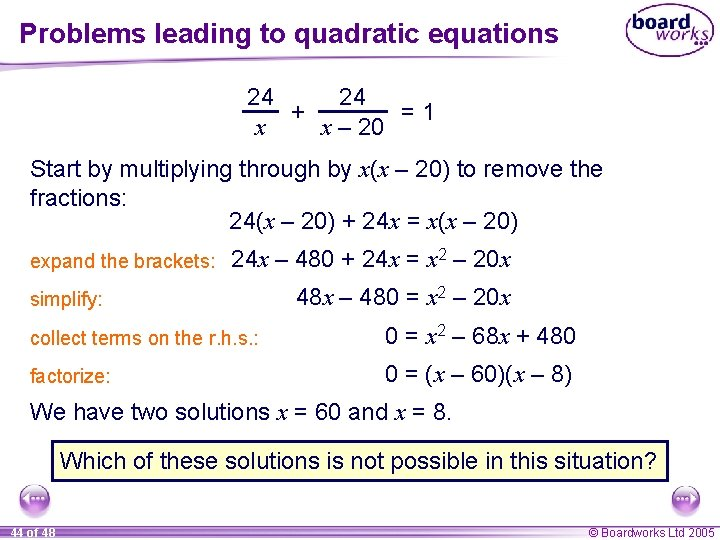Problems leading to quadratic equations 24 24 + =1 x x – 20 Start by multiplying through by x(x – 20) to remove the fractions: 24(x – 20) + 24 x = x(x – 20) expand the brackets: 24 x – 480 + 24 x = x 2 – 20 x simplify: 48 x – 480 = x 2 – 20 x collect terms on the r. h. s. : 0 = x 2 – 68 x + 480 factorize: 0 = (x – 60)(x – 8) We have two solutions x = 60 and x = 8. Which of these solutions is not possible in this situation? 44 of 48 © Boardworks Ltd 2005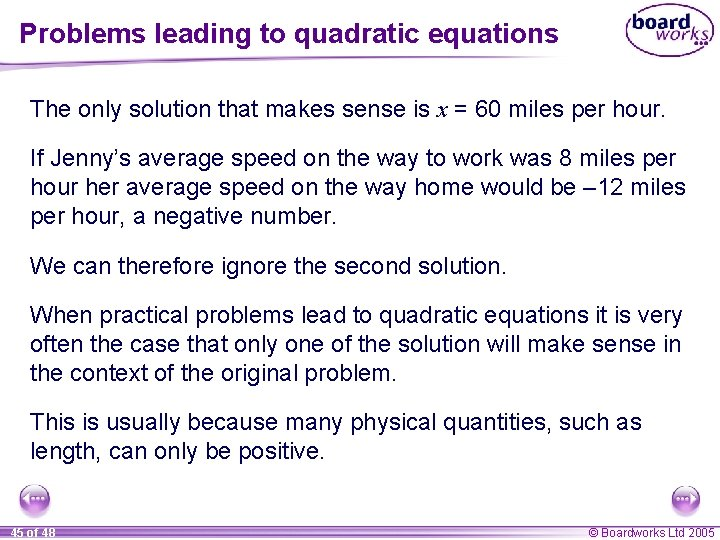Problems leading to quadratic equations The only solution that makes sense is x = 60 miles per hour. If Jenny’s average speed on the way to work was 8 miles per hour her average speed on the way home would be – 12 miles per hour, a negative number. We can therefore ignore the second solution. When practical problems lead to quadratic equations it is very often the case that only one of the solution will make sense in the context of the original problem. This is usually because many physical quantities, such as length, can only be positive. 45 of 48 © Boardworks Ltd 2005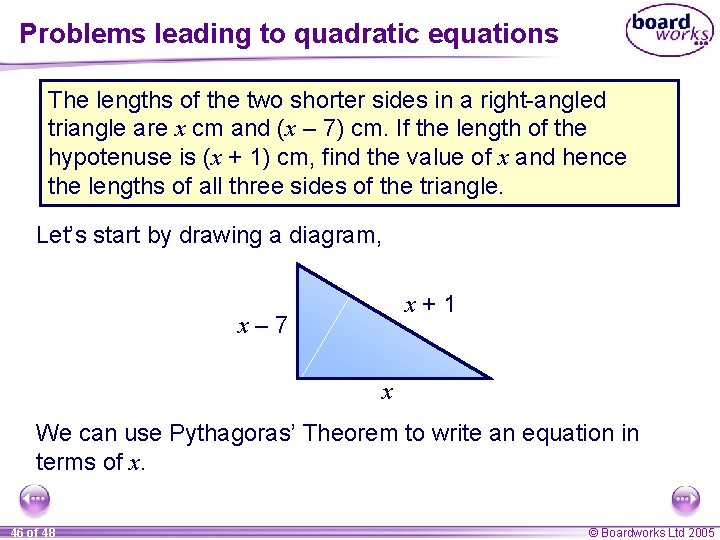Problems leading to quadratic equations The lengths of the two shorter sides in a right-angled triangle are x cm and (x – 7) cm. If the length of the hypotenuse is (x + 1) cm, find the value of x and hence the lengths of all three sides of the triangle. Let’s start by drawing a diagram, x+1 x– 7 x We can use Pythagoras’ Theorem to write an equation in terms of x. 46 of 48 © Boardworks Ltd 2005Problems leading to quadratic equations The lengths of the two shorter sides in a right-angled triangle are x cm and (x – 7) cm. If the length of the hypotenuse is (x + 1) cm, find the value of x and hence the lengths of all three sides of the triangle. x 2 + (x – 7)2 = (x + 1)2 x 2 + (x – 7) = (x + 1) expand: x 2 + x 2 – 7 x + 49 = x 2 + x + 1 simplify: collect on the l. h. s. : factorize: 2 x 2 – 14 x + 49 = x 2 + 2 x + 1 x 2 – 16 x + 48 = 0 (x – 4)(x – 12) = 0 x=4 47 of 48 or x = 12 © Boardworks Ltd 2005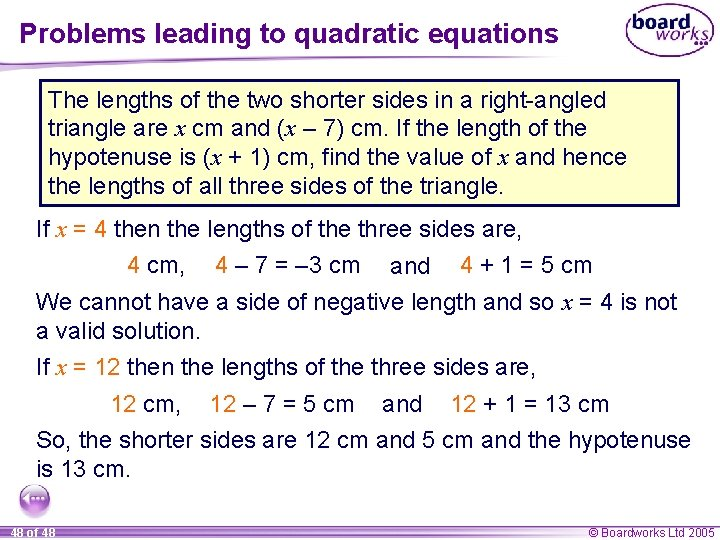Problems leading to quadratic equations The lengths of the two shorter sides in a right-angled triangle are x cm and (x – 7) cm. If the length of the hypotenuse is (x + 1) cm, find the value of x and hence the lengths of all three sides of the triangle. If x = 4 then the lengths of the three sides are, 4 cm, 4 – 7 = – 3 cm and 4 + 1 = 5 cm We cannot have a side of negative length and so x = 4 is not a valid solution. If x = 12 then the lengths of the three sides are, 12 cm, 12 – 7 = 5 cm and 12 + 1 = 13 cm So, the shorter sides are 12 cm and 5 cm and the hypotenuse is 13 cm. 48 of 48 © Boardworks Ltd 2005